concept in machine learning – `Boosting`.

All algorithms are implemented in Python.

There are two main tasks which people want to finish with Machine Learning.

• Classification
• Regression

There are a lot of other ways to do it but now we focus on `boosting` algorithm. You know that it’s a fantastic way to make our work done.

If you never hear about adaboost, I recommend you to finish the 7-th lab in MIT 6.034. It will help you a lot to understand what I’m taking about. But this lab didn’t build adaboost completely. So, I implement it individually.

Give the input training samples which have tag with it.where x[i] is the feature of the i-th sample point and y[i] is the `label` (soemtimes we call it as `tag`) with the sample point.

In this algorithm, there are only two different label of samples {-1, +1}.

Some classifier like decision tree also can work correctly about classification. But it’s also easy to overfitting. So, we can’t use it in some special situation. Instread of using decision tree, we use `decision stump` which is a special type of decision tree which’s depth is only one. So we call it as `decision stump`.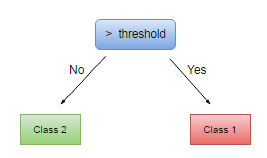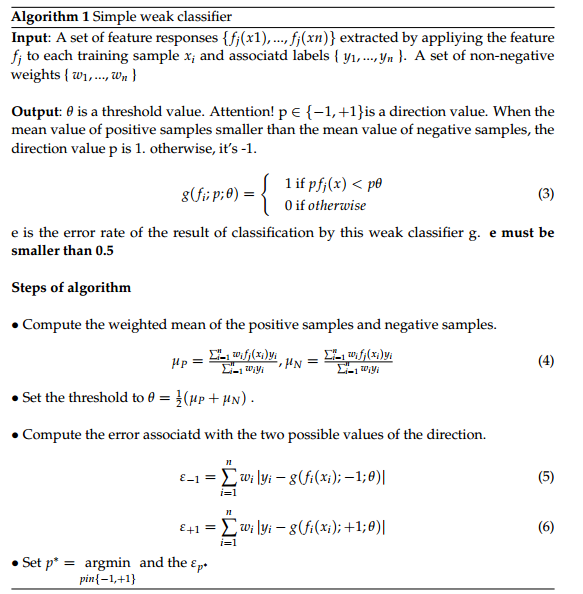`Yoav Freund` and `Robert Schapire` create this algorithm AdaBoost which means adaptive boosting.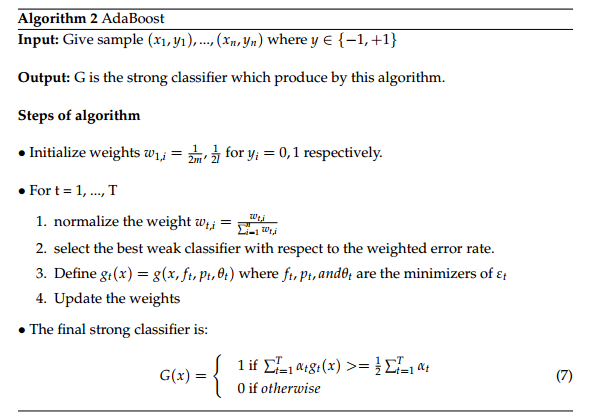Test case:

There are training points with two different label. What if we input a point which’s type is unkown, what the result will be?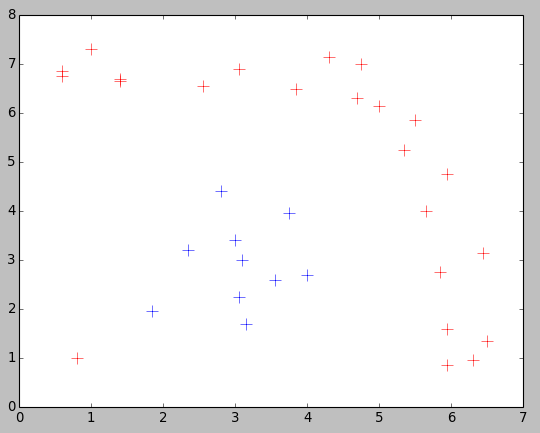The test result is below there: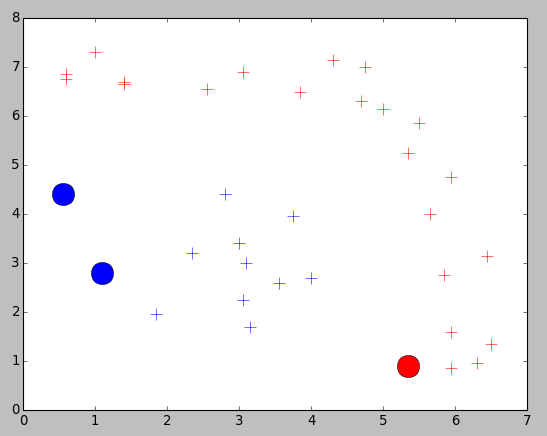Just create a object of class `Adaboost` with your training samples with label. like this:

API `prediction()` of class AdaBoost will return the result of prediction according to the model. All job done.

You could find other test case in my repository in github.

There is an assignment about AdaBoost in Stanford CS 229, which will ask student to implement stump booster. But I don’t really understand the skeleton of that source code. I think there must be something worng with that matlab script `stump_booster.m`. The week classifier can’t lost the direction information.

Run `boost_example.m`, you will see the classifier line with different iteration.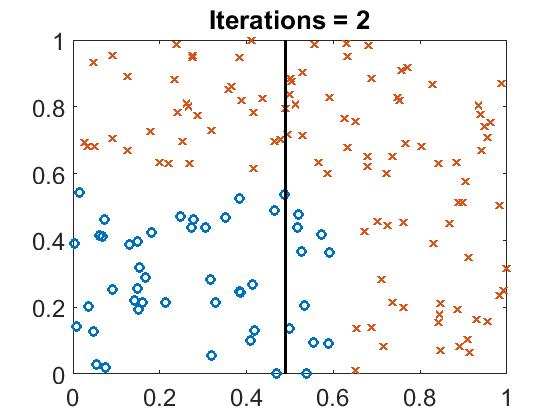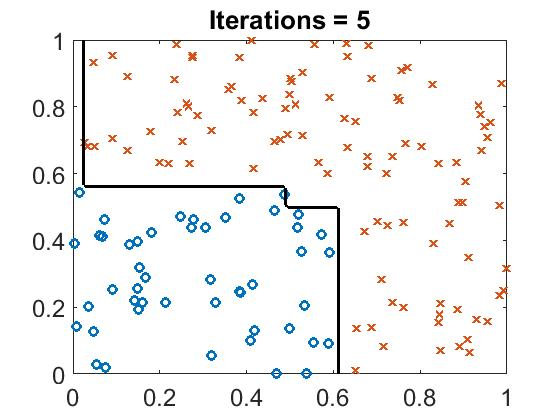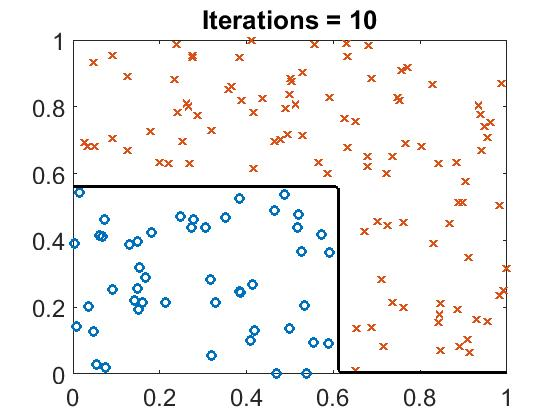### Boosting Tree

We have knew to use `AdaBoost` to do classification. `Boosting Tree` will help us to do regression.

We also use decision stump as the weak classifier. But implementation of decision stump in this algorithm is not the same as that in AdaBoost.

There are ten samples in my test module:

The expected value of Original_Data[i] is ExpVal[i]. The input is from 1 to 10. How about to predict the output when the input is 1 or 11?

Let’s test it. Here is the result: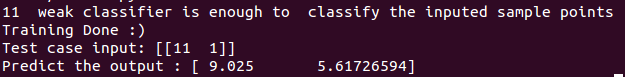Just used 11 weak classifier to construct a stronger classifier to do the regressio. The output is reasonable.

Here is my implementation of `Boosting Tree`
Implementation of Boosting Tree in Python

Reference:

1. MIT-6.034, Artificial Intelligence. Lab-7
2. << The statistic methods >> by HangLi.
3. Wikipedia

Photo by Jason Leaster